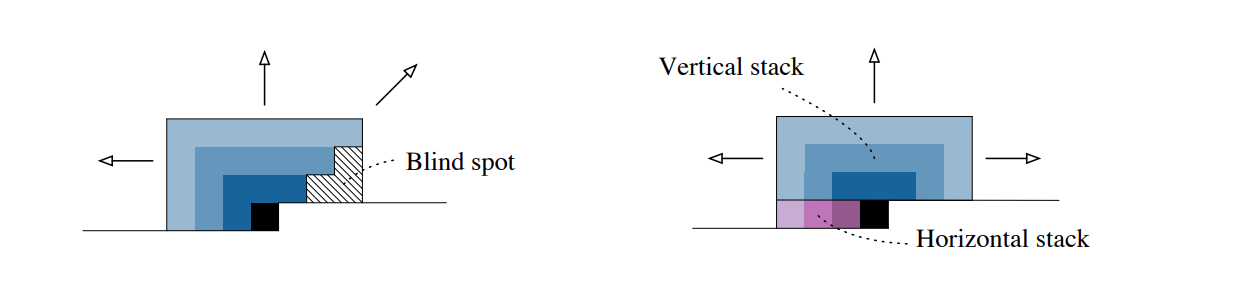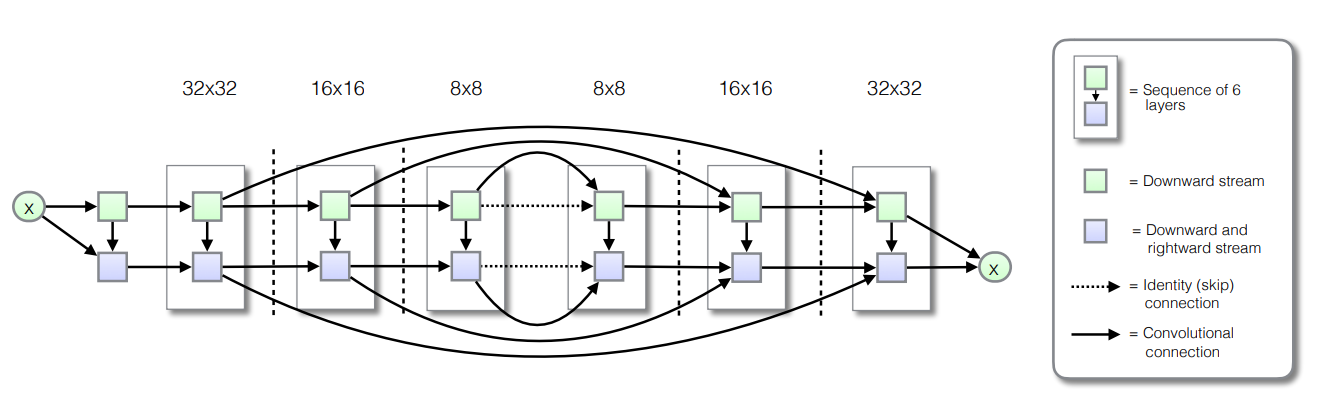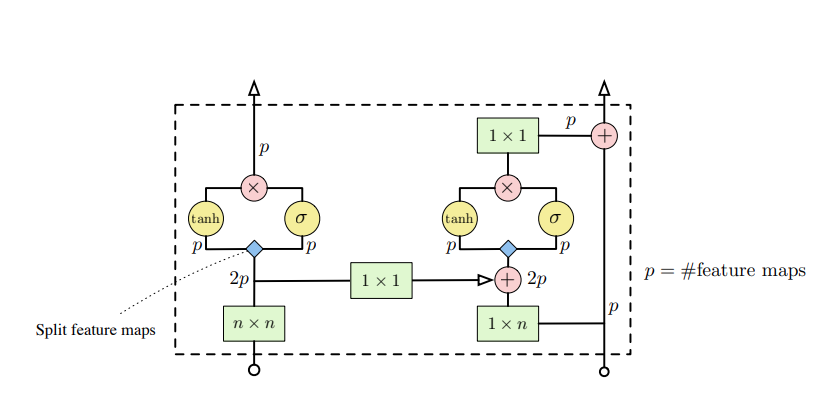# Easy PixelCNN with tfprobability

PixelCNN is a deep learning architecture - or bundle of architectures - designed to generate highly realistic-looking images. To use it, no reverse-engineering of arXiv papers or search for reference implementations is required: TensorFlow Probability and its R wrapper, tfprobability, now include a PixelCNN distribution that can be used to train a straightforwardly-defined neural network in a parameterizable way.

Sigrid Keydana (RStudio)https://www.rstudio.com/
05-29-2020

We’ve seen quite a few examples of unsupervised learning (or self-supervised learning, to choose the more correct but less popular term) on this blog.

Often, these involved Variational Autoencoders (VAEs), whose appeal lies in them allowing to model a latent space of underlying, independent (preferably) factors that determine the visible features. A possible downside can be the inferior quality of generated samples. Generative Adversarial Networks (GANs) are another popular approach. Conceptually, these are highly attractive due to their game-theoretic framing. However, they can be difficult to train. PixelCNN variants, on the other hand – we’ll subsume them all here under PixelCNN – are generally known for their good results. They seem to involve some more alchemy1 though. Under those circumstances, what could be more welcome than an easy way of experimenting with them? Through TensorFlow Probability (TFP) and its R wrapper, tfprobability, we now have such a way.

This post first gives an introduction to PixelCNN, concentrating on high-level concepts (leaving the details for the curious to look them up in the respective papers). We’ll then show an example of using tfprobability to experiment with the TFP implementation.

## PixelCNN principles

### Autoregressivity, or: We need (some) order

The basic idea in PixelCNN is autoregressivity. Each pixel is modeled as depending on all prior pixels. Formally:

$p(\mathbf{x}) = \prod_{i}p(x_i|x_0, x_1, ..., x_{i-1})$

Now wait a second - what even are prior pixels? Last I saw one images were two-dimensional. So this means we have to impose an order on the pixels. Commonly this will be raster scan order: row after row, from left to right. But when dealing with color images, there’s something else: At each position, we actually have three intensity values, one for each of red, green, and blue. The original PixelCNN paper carried through autoregressivity here as well, with a pixel’s intensity for red depending on just prior pixels, those for green depending on these same prior pixels but additionally, the current value for red, and those for blue depending on the prior pixels as well as the current values for red and green.

$p(x_i|\mathbf{x}<i) = p(x_{i,R}|\mathbf{x}<i)\ p(x_{i,G}|\mathbf{x}<i, x_{i,R})\ p(x_{i,B}|\mathbf{x}<i, x_{i,R}, x_{i,G})$

Here, the variant implemented in TFP, PixelCNN++ , introduces a simplification; it factorizes the joint distribution in a less compute-intensive way.2

Technically, then, we know how autoregressivity is realized; intuitively, it may still seem surprising that imposing a raster scan order “just works” (to me, at least, it is). Maybe this is one of those points where compute power successfully compensates for lack of an equivalent of a cognitive prior.

### Masking, or: Where not to look

Now, PixelCNN ends in “CNN” for a reason – as usual in image processing, convolutional layers (or blocks thereof) are involved. But – is it not the very nature of a convolution that it computes an average of some sorts, looking, for each output pixel, not just at the corresponding input but also, at its spatial (or temporal) surroundings? How does that rhyme with the look-at-just-prior-pixels strategy?

Surprisingly, this problem is easier to solve than it sounds. When applying the convolutional kernel, just multiply with a mask that zeroes out any “forbidden pixels” – like in this example for a 5x5 kernel, where we’re about to compute the convolved value for row 3, column 3:

$\left[\begin{array} {rrr} 1 & 1 & 1 & 1 & 1\\ 1 & 1 & 1 & 1 & 1\\ 1 & 1 & 1 & 0 & 0\\ 0 & 0 & 0 & 0 & 0\\ 0 & 0 & 0 & 0 & 0\\ \end{array}\right]$

This makes the algorithm honest, but introduces a different problem: With each successive convolutional layer consuming its predecessor’s output, there is a continuously growing blind spot (so-called in analogy to the blind spot on the retina, but located in the top right) of pixels that are never seen by the algorithm. Van den Oord et al. (2016) fix this by using two different convolutional stacks, one proceeding from top to bottom, the other from left to right3.Fig. 1: Left: Blind spot, growing over layers. Right: Using two different stacks (a vertical and a horizontal one) solves the problem. Source: van den Oord et al., 2016.

### Conditioning, or: Show me a kitten

So far, we’ve always talked about “generating images” in a purely generic way. But the real attraction lies in creating samples of some specified type – one of the classes we’ve been training on, or orthogonal information fed into the network. This is where PixelCNN becomes Conditional PixelCNN, and it is also where that feeling of magic resurfaces. Again, as “general math” it’s not hard to conceive. Here, $$\mathbf{h}$$ is the additional input we’re conditioning on:

$p(\mathbf{x}| \mathbf{h}) = \prod_{i}p(x_i|x_0, x_1, ..., x_{i-1}, \mathbf{h})$

But how does this translate into neural network operations? It’s just another matrix multiplication ($$V^T \mathbf{h}$$) added to the convolutional outputs ($$W \mathbf{x}$$).

$\mathbf{y} = tanh(W_{k,f} \mathbf{x} + V^T_{k,f} \mathbf{h}) \odot \sigma(W_{k,g} \mathbf{x} + V^T_{k,g} \mathbf{h})$

(If you’re wondering about the second part on the right, after the Hadamard product sign – we won’t go into details, but in a nutshell, it’s another modification introduced by , a transfer of the “gating” principle from recurrent neural networks, such as GRUs and LSTMs, to the convolutional setting.)

So we see what goes into the decision of a pixel value to sample. But how is that decision actually made?

### Logistic mixture likelihood , or: No pixel is an island

Again, this is where the TFP implementation does not follow the original paper, but the latter PixelCNN++ one. Originally, pixels were modeled as discrete values, decided on by a softmax over 256 (0-255) possible values. (That this actually worked seems like another instance of deep learning magic. Imagine: In this model, 254 is as far from 255 as it is from 0.)

In contrast, PixelCNN++ assumes an underlying continuous distribution of color intensity, and rounds to the nearest integer. That underlying distribution is a mixture of logistic distributions, thus allowing for multimodality:

$\nu \sim \sum_{i} \pi_i \ logistic(\mu_i, \sigma_i)$

### Overall architecture and the PixelCNN distribution

Overall, PixelCNN++, as described in , consists of six blocks. The blocks together make up a UNet-like structure, successively downsizing the input and then, upsampling again:Fig. 2: Overall structure of PixelCNN++. From: Salimans et al., 2017.

In TFP’s PixelCNN distribution, the number of blocks is configurable as num_hierarchies, the default being 3.

Each block consists of a customizable number of layers, called ResNet layers due to the residual connection (visible on the right) complementing the convolutional operations in the horizontal stack:Fig. 3: One so-called "ResNet layer", featuring both a vertical and a horizontal convolutional stack. Source: van den Oord et al., 2017.

In TFP, the number of these layers per block is configurable as num_resnet.

num_resnet and num_hierarchies are the parameters you’re most likely to experiment with, but there are a few more you can check out in the documentation. The number of logistic distributions in the mixture is also configurable, but from my experiments it’s best to keep that number rather low to avoid producing NaNs during training.

Let’s now see a complete example.

## End-to-end example

Our playground will be QuickDraw, a dataset – still growing – obtained by asking people to draw some object in at most twenty seconds, using the mouse. (To see for yourself, just check out the website). As of today, there are more than a fifty million instances, from 345 different classes.

First and foremost, these data were chosen to take a break from MNIST and its variants. But just like those (and many more!), QuickDraw can be obtained, in tfdatasets-ready form, via tfds, the R wrapper to TensorFlow datasets. In contrast to the MNIST “family” though, the “real samples” are themselves highly irregular, and often even missing essential parts. So to anchor judgment, when displaying generated samples we always show eight actual drawings with them.

### Preparing the data

The dataset being gigantic, we instruct tfds to load the first 500,000 drawings “only.”

library(reticulate)

# >= 2.2 required
library(tensorflow)
library(keras)

# make sure to use at least version 0.10
library(tfprobability)

library(tfdatasets)
# currently to be installed from github
library(tfds)

# load just the first 500,000 images
# ... be prepared for this to take some time
train_ds <- tfds_load("quickdraw_bitmap", split='train[:500000]')

To speed up training further, we then zoom in on twenty classes. This effectively leaves us with ~ 1,100 - 1,500 drawings per class.

# bee, bicycle, broccoli, butterfly, cactus,
# frog, guitar, lightning, penguin, pizza,
# rollerskates, sea turtle, sheep, snowflake, sun,
# swan, The Eiffel Tower, tractor, train, tree
classes <- c(26, 29, 43, 49, 50,
125, 134, 172, 218, 225,
246, 255, 258, 271, 295,
296, 308, 320, 322, 323
)

classes_tensor <- tf$cast(classes, tf$int64)

train_ds <- train_ds %>%
dataset_filter(
function(record) tf$reduce_any(tf$equal(classes_tensor, record$label), -1L) ) The PixelCNN distribution expects values in the range from 0 to 255 – no normalization required. Preprocessing then consists of just casting pixels and labels each to float: preprocess <- function(record) { record$image <- tf$cast(record$image, tf$float32) record$label <- tf$cast(record$label, tf$float32) list(tuple(record$image, record$label)) } batch_size <- 32 train <- train_ds %>% dataset_map(preprocess) %>% dataset_shuffle(10000) %>% dataset_batch(batch_size) ### Creating the model We now use tfd_pixel_cnn to define what will be the loglikelihood used by the model. dist <- tfd_pixel_cnn( image_shape = c(28, 28, 1), conditional_shape = list(), num_resnet = 5, num_hierarchies = 3, num_filters = 128, num_logistic_mix = 5, dropout_p =.5 ) image_input <- layer_input(shape = c(28, 28, 1)) label_input <- layer_input(shape = list()) log_prob <- dist %>% tfd_log_prob(image_input, conditional_input = label_input) This custom loglikelihood is added as a loss to the model, and then, the model is compiled with just an optimizer specification only. During training, loss first decreased quickly, but improvements from later epochs were smaller. model <- keras_model(inputs = list(image_input, label_input), outputs = log_prob) model$add_loss(-tf$reduce_mean(log_prob)) model$compile(optimizer = optimizer_adam(lr = .001))

model %>% fit(train, epochs = 10)

To jointly display real and fake images:

for (i in classes) {

real_images <- train_ds %>%
dataset_filter(
function(record) record$label == tf$cast(i, tf$int64) ) %>% dataset_take(8) %>% dataset_batch(8) it <- as_iterator(real_images) real_images <- iter_next(it) real_images <- real_images$image %>% as.array()
real_images <- real_images[ , , , 1]/255

generated_images <- dist %>% tfd_sample(8, conditional_input = i)
generated_images <- generated_images %>% as.array()
generated_images <- generated_images[ , , , 1]/255

images <- abind::abind(real_images, generated_images, along = 1)
png(paste0("draw_", i, ".png"), width = 8 * 28 * 10, height = 2 * 28 * 10)
par(mfrow = c(2, 8), mar = c(0, 0, 0, 0))
images %>%
purrr::array_tree(1) %>%
purrr::map(as.raster) %>%
purrr::iwalk(plot)
dev.off()
}

From our twenty classes, here’s a choice of six, each showing real drawings in the top row, and fake ones below.

We probably wouldn’t confuse the first and second rows, but then, the actual human drawings exhibit enormous variation, too. And no one ever said PixelCNN was an architecture for concept learning. Feel free to play around with other datasets of your choice – TFP’s PixelCNN distribution makes it easy.

## Wrapping up

In this post, we had tfprobability / TFP do all the heavy lifting for us, and so, could focus on the underlying concepts. Depending on your inclinations, this can be an ideal situation – you don’t lose sight of the forest for the trees. On the other hand: Should you find that changing the provided parameters doesn’t achieve what you want, you have a reference implementation to start from. So whatever the outcome, the addition of such higher-level functionality to TFP is a win for the users. (If you’re a TFP developer reading this: Yes, we’d like more :-)).

To everyone though, thanks for reading!

Oord, Aaron van den, Nal Kalchbrenner, and Koray Kavukcuoglu. 2016. “Pixel Recurrent Neural Networks.” CoRR abs/1601.06759. http://arxiv.org/abs/1601.06759.
Oord, Aaron van den, Nal Kalchbrenner, Oriol Vinyals, Lasse Espeholt, Alex Graves, and Koray Kavukcuoglu. 2016. “Conditional Image Generation with PixelCNN Decoders.” CoRR abs/1606.05328. http://arxiv.org/abs/1606.05328.
Salimans, Tim, Andrej Karpathy, Xi Chen, and Diederik P. Kingma. 2017. “PixelCNN++: A PixelCNN Implementation with Discretized Logistic Mixture Likelihood and Other Modifications.” In ICLR.

1. Alluding to Ali Rahimi’s (in)famous “deep learning is alchemy” talk at NeurIPS 2017. I would suspect that to some degree, that statement resonates with many DL practitioners – although one need not agree that more mathematical rigor is the solution.↩︎

2. For details, see .↩︎

3. .For details, see .↩︎

### Reuse

Text and figures are licensed under Creative Commons Attribution CC BY 4.0. The figures that have been reused from other sources don't fall under this license and can be recognized by a note in their caption: "Figure from ...".

### Citation

Keydana (2020, May 29). Posit AI Blog: Easy PixelCNN with tfprobability. Retrieved from https://blogs.rstudio.com/tensorflow/posts/2020-05-29-pixelcnn/
@misc{keydanapixelcnn,
}